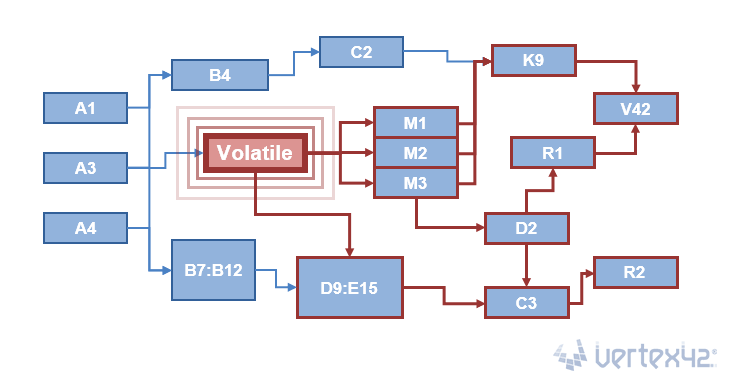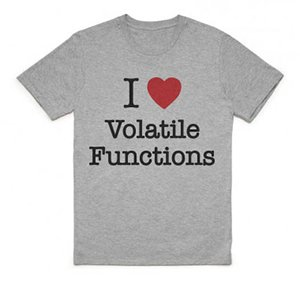Volatile Functions – What’s the Big Deal?

A Volatile Function in a spreadsheet is a function like RAND, TODAY, NOW or OFFSET that must be recalculated every time any cell is changed and every other time that Excel recalculates. At each recalculation, all volatile functions fire off and recalculate, setting up a chain reaction of recalculation of every cell that might depend on them.We had some fun creating the above image to demonstrate the effect of a volatile function. This is simplifying things quite a bit, but the point is that when a cell changes, every cell that refers to it must also be updated. So, volatile functions usually only cause performance issues if a lot of other cells depend on them.

There are some volatile functions like RAND, RANDBETWEEN, NOW, and TODAY that are obviously intended to cause a recalculation every time. But, you may not be aware that OFFSET and INDIRECT are also volatile functions, even though it might not seem necessary for them to recalculate. The functions INFO and CELL can also be volatile, depending on their arguments.

Why are OFFSET and INDIRECT Volatile Functions?

Excel uses something called "Smart Calculation" to only recalculate the cells that need to be recalculated. It keeps track of all references and formulas and uses a dependency tree to figure out which cells need to be recalculated (and in what order) when changes are made to the spreadsheet. OFFSET and INDIRECT create references on the fly, so Excel must recalculate them any time the worksheet changes, just in case a cell that they are referring to was changed.

See the list of references at the end of this article if you want to learn more about how calculation works in Excel and how to optimize calculation speed.

But my computer is fast, so what's the big deal?It might not be a big deal at all! Volatile functions are not inherently bad. I think they get a bad rap partly because they are called volatile - as if some explosion was about to go off in your spreadsheet. In fact, most of the volatile functions allow you to do so some amazingly awesome things!

I am a fan of volatile functions. If I actually had a shirt like the one my brother designed on the right, I would wear it. I love OFFSET. I love TODAY. I love INDIRECT. I love RAND. I love RANDBETWEEN. I hardly ever use NOW or INFO or CELL, but I bet if I used them more I would love them too.

The volatile function itself takes only a tiny fraction of a second to calculate, such as using TODAY to display the date at the top of your worksheet. So, you don't usually see problems until there are hundreds of thousands of calculations that depend on the result of the volatile function, or if you are using volatile functions in too many formulas.

Am I suggesting you fill your spreadsheet with volatile functions? Sure, if that would be cool and would allow you to do something awesome. But that's not my point. Use volatile formulas if you need them. If you run into speed problems, look for inefficiencies and fix them, but don't allow your fear of volatile functions or other people's fear prevent you from using them to do cool things with your spreadsheets.

Some Examples of Why I Love Volatile Functions

The TODAY function

I love using TODAY in project schedules, invoices, purchase orders, and other forms and reports where you want to display the date that the form or report was printed.

Another fun use for TODAY is within conditional formatting rules to highlight the current date in a calendar.You can either use a formula like =B11=TODAY() for the conditional formatting rule, or you can use the built-in rule shown in the image below.NOTEConditional formatting rules are already volatile in their own way. The formulas used in conditional formatting must be recalculated for the portion of the screen that is currently displayed (whether or not you use volatile formulas for the rules). That is usually only a problem if the formulas used for the rules are not efficient. When conditional formatting is the culprit (rather than volatile functions), and there is no way to improve efficiency of the rules, you can speed up the response of the worksheet by zooming in while editing (so that less of the formatted range is displayed).

The RAND and RANDBETWEEN functions

RAND returns a random number between and including 0 and 1. RANDBETWEEN returns a random whole number between and including two numbers. Pressing F9 will force a recalculation and the results will change ... randomly.

Below are a couple of formulas for generating random numbers from specific distributions. See my Monte Carlo Simulation template for a larger variety of ways to generate random numbers for simulation models.

1. Generate a Random Number from a Uniform Distribution

`=RAND()*(max_value-min_value)+min_value`

2. Generate a Random Number from a Normal Distribution

`=NORMINV(RAND(),mean,standard_dev)`

Now for the fun: If you generate random rates of return, you can simulate the change in value of an investment over time. Pressing F9 makes the graph change. The animated GIF below shows what this can look like:This example is included in the download file, and the technique is also used in my Savings Calculator and 401k Calculator.

The OFFSET function

`=OFFSET(reference,offset_rows,offset_cols,[height],[width])`

1. Dynamic Named Ranges

I have already written about using OFFSET for dynamic drop-down lists, but I'll mention it again here because it's one of the main reasons I love OFFSET.

2. Robust Running Balances

I like using OFFSET(current_cell,-1,0) to reference a cell immediately above the current one. This makes it possible to create running balances and running counts that are more robust to user actions such as sorting, deleting, inserting, or moving rows within the table.

The example below shows the result of deleting a row within a table that uses a running count and running balance. The formulas that use OFFSET don't result in #REF! errors.NOTE This is an example of a very efficient use of OFFSET, but you can accomplish the same thing without a volatile function. You can define a relative named range like prevBalance (by using a relative reference of \$I77 in the Refers To field) and use the formula =prevBalance+H78.

The INDIRECT function

`=INDIRECT(ref_text,[a1])`

1. Dependent Drop-Down Lists

I use INDIRECT in some of my spreadsheets to create dependent drop-down lists. The details for how to do that can be found in my article "Create a Drop Down List in Excel".

2. Including the Worksheet Name in a Dynamic Reference

This is a technique commonly used in dashboard reports to summarize data from multiple worksheets that have a similar structure. The example below sums the sales in column B for the worksheets named Jan, Feb, Mar, and Apr.If the worksheet name includes a space, then you need to enclose the name in apostrophes like this:

`=SUM(INDIRECT("'"&worksheet_name&"'!B:B"))`

It's Not Always the Volatile Function's Fault

If you have a complex and inefficient spreadsheet, dependence on one or more volatile functions might be a cause for the slow calculation, but they aren't always the cause. Below are a couple examples of inefficient formulas that are NOT volatile:

1. Using SUM(\$A\$1:\$A50) for a cumulative sum. Yes, it's easy, but the number of calculations goes up exponentially as the number of rows increases. It's better to use =next_value+previous_sum or =SUM(next_value,previous_sum) instead. For example, if column B contains the cumulative sum of column A, and row 1 contains labels, enter =SUM(A2,B1) in cell B2 and copy the formula down.
2. Using SUMPRODUCT or an Array Formula on entire columns such as =SUMPRODUCT(A:A,1*(A:A>5),1*(A:A<20)). SUMIFS and many other built-in functions are designed to ignore unused cells, but array formulas do not.

Volatile functions in drop-down lists (No Big Deal?)

Except in Excel Online, I have not come across a situation where using OFFSET or INDIRECT causes performance problems (i.e. slow calculation) when used for dynamic ranges in drop-down lists. When you select a value from a drop-down list, the cell stores it as a single value, not a formula. In theory, there is no need for the Source of a drop-down list to be included in the dependency tree. It only needs to be recalculated when the user accesses the drop-down.

The named range containing a volatile function may be recalculated after every change to the spreadsheet, but if you only have a few of these named ranges, and you only use them for drop-down lists, then no big deal.

To test this theory, I created a spreadsheet that used over 100,000 inefficient cumulative sum formulas of the type SUM(A\$5:A400), resulting in about 300 million addition operations, all depending on each other in series, beginning with a single start cell. There was about a 5 second delay in calculation when the start cell was modified (even a 1 second delay in calculation gets annoying). I then added data validation drop-down lists to all 100k cells, each with a formula like =OFFSET(A6,0,0,RANDBETWEEN(1,50)) so that the drop-down lists were doubly volatile (OFFSET using RANDBETWEEN as an argument). These 100,000 drop-down lists did not noticeably affect the calculation speed at all. I suspect the reason is that the formula used for the Source in the drop-down list is not evaluated until you click on the drop-down (that would make sense after all).

Excel Online: There does appear to be a problem when using volatile functions for drop-down lists in Excel Online.

What do You Think?

If you have any strong opinions about volatile functions, or have any examples of ways that you use them to do cool things, please share by leaving a comment below.

References

There are a lot of great articles written by experts that provides tips for improving calculation efficiency in your spreadsheet models. Some of these are listed below.

• I like the reference to V42 and R2 D2 in the main image for the article. I helped make the image and didn’t even realize those were in there :-)

• I am passionate about excel and see an article like this, explaining a step like playing, I confess that makes me stop here at work for hours. Congratulations Jon Wittwer for writing an article with such rich detail. You are here on the blog Vertex are beasts. I love reading the articles here.
Thank you for always sharing good things.

• Hello.
First off, thank you for a greatly instructive and well written article.
Now, pardon my ignorance, but would you please explain how to achieve “=next_value+previous_sum” in “real formula language”?
Cheers!
P.S. – Will I be notified by email once an answer is posted? :D

• @Alphonse … This means that if the column you are summing is column B and the cumulative sum is in column C, the formula in C3 would be =B3+C2. Or, you could use C2=SUM(B2,C1) and copy the formula down (and this works even if row 1 contains column labels). I updated the article to include an example.

• So, if I understood correctly, it is best to create and use subtotals along the way, than to total up a whole bunch of individual values… so I imagine it would look something like this:
C2=SUM(B2,C1), C3=SUM(B3,C2), C4=SUM(B4,C3), or at whatever interval might be necessary.
Thank you for your explanation and example which I will put into action, and thank you again for a great article.
Cheers!

• @Alphonse… “best” is relative to what you are wanting to do with your spreadsheet of course. Although C2=SUM(B2,C1) might be more efficient for a cumulative sum, it would not work well if you were planning to delete or move rows around. As the title “what’s the big deal?” suggests, it may not matter whether you are using the most efficient formulas for the job.

• the sales forecast model description is excellent.

the ‘asides’ such as volatile functions description are a generous bonus and not readily found elsewhere. Thank you.